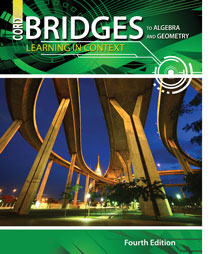# Bridges to Algebra and Geometry - 4th Edition

## Chapter 5: Rational Numbers

Some links are repeated for use with more than one lesson.

### 5.1 Equivalent Fractions

http://www.explorelearning.com/index.cfm?method=cResource.dspResourcesForCourse&CourseID=212
This site has examples and problems for the students to solve that deal with fractions.

http://www.math.com/school/subject1/lessons/S1U4L2GL.html
This site has the rules and examples of reducing fractions.

http://www.cut-the-knot.org/arithmetic/frac_div.shtml
This site has examples and the definition of equivalent fractions.

### 5.2 Fractions and Decimals

http://www.explorelearning.com/index.cfm?method=cResource.dspResourcesForCourse&CourseID=212
This site has examples and problems for the students to solve that deal with fractions.

http://www.mathsisfun.com/converting-fractions-decimals.html
NEW This site shows how to convert fractions to decimals.

http://www.sosmath.com/algebra/fraction/frac6/frac6.html
NEW This site explains how to convert decimals to fractions and gives students problems to practice this concept.

This site shows how to construct a table of conversions from fractions to decimals.

### 5.3 Comparing and Ordering Rational Numbers and Inequalities

http://www.aplusmath.com/Flashcards/index.html
This site has practice problems for students on addition, subtraction, multiplication, and division with rational numbers.

### 5.4 Adding and Subtracting Rational Numbers

NEW This site describes the steps to add fractions, including practice problems.

http://www.mathsisfun.com/fractions_subtraction.html
NEW This site describes the steps to subtract fractions, including practice problems.

http://www.regentsprep.org/Regents/math/ALGEBRA/AV5/Fequations.htm
NEW This site shows how to solve equations with fractional terms and operations.

http://www.regentsprep.org/Regents/math/ALGEBRA/AV5/Pequations.htm
NEW This page has practice problems for solving equations with fractional terms and operations.

http://www.aplusmath.com/Flashcards/index.html
This site has practice problems for students on addition, subtraction, multiplication, and division with rational numbers.

http://www.math.com/school/subject1/lessons/S1U4L3GL.html
This site has the rules and examples of adding and subtracting fractions.

### 5.5 Multiplying Rational Numbers

http://www.mathsisfun.com/fractions_multiplication.html
NEW This site describes the steps to multiply fractions, including practice problems.

http://www.regentsprep.org/Regents/math/ALGEBRA/AV5/multdivide.htm
NEW This page presents the techniques for multiplying fractions with numbers and variables.

http://www.aplusmath.com/Flashcards/index.html
This site has practice problems for students on addition, subtraction, multiplication, and division with rational numbers.

http://www.math.com/school/subject1/lessons/S1U4L4GL.html
This site has the rules and examples of multiplying fractions.

### 5.6 Dividing Rational Numbers

http://www.mathsisfun.com/fractions_division.html
NEW This site describes the steps to divisde fractions, including practice problems.

http://www.coolmath4kids.com/fractions/fractions-16-dividing-01.html
NEW This site has a couple pages that show how to divide fractions.

http://www.aplusmath.com/Flashcards/index.html
This site has practice problems for students on addition, subtraction, multiplication, and division with rational numbers.

http://www.math.com/school/subject1/lessons/S1U4L5GL.html
This site has the rules and examples of dividing fractions.

### 5.7 Solving Inequalities with Addition and Subtraction

http://www.mathsisfun.com/algebra/inequality-properties.html
NEW This page covers the several properties of inequalities, including some practice problems.

### 5.8 Solving Inequalities with Multiplication and Division

http://statisticslectures.com/topics/multiplydivideinequalities/
NEW This page summarizes the multiplication and division properties of inequalities as they are used to solve for an unknown variable, including a short video explanation.